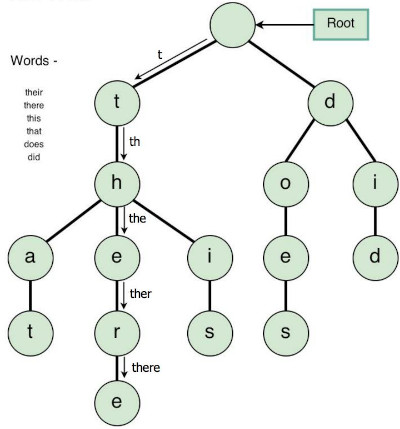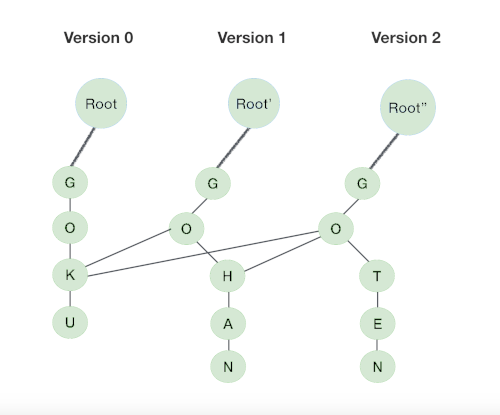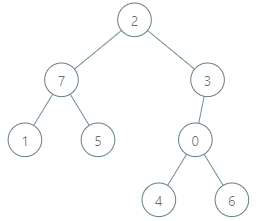×

Search anything:

# Persistent Trie

#### Data Structures trie String AlgorithmsFREE BOOK -> Problems for the day before your Coding Interview (on Amazon)

In this article, we discuss the Trie data structure and how to make it persistent to solve various problems optimally.

1. What is a Trie?
2. Persistency of a data structure
3. Persistent Trie
4. Time and Space Complexity Analysis
5. Comparison between basic and persistent trie
6. Example Problem

Pre-requisites:

## What is a Trie?

This is a special tree that can store strings in an ordered efficient way.
We can visualize it as a graph, that is each node has a maximum of 26 children(26 letters of aplhabet) and edges connect each parent node to its children.
The root node will therefore contain 26 outgoing edges.
It allows for efficient retrieval of data, which optimizes search complexities O(string length) unlike trees O(mlogn) where M is the max string length, n is the keys in the tree.
An example of a trie is shown below;### Trie Operations.

Node declaration

``````class TrieNode{
bool isEndOfString;
TrieNode children[];

TrieNode(){
isEndOfString = false;
children = new TrieNode;
}
}
``````

Node initialization

``````TrieNode root;

class Trie{
public:
root = new TrieNode();
}
``````

Insertion
We start from the root node and search for a reference corresponding to the first character of the string we want to insert.
If a reference exists, we traverse down the trie following the reference to the next children level.
If not, we create a new node and refer it with parent's reference matching the current string character. This is done repeatedly until we get to the last character of the string. We mark the last character as an end node and terminate.

``````void insert(string str){
TrieNode node = root;
for(char c in string str){
if (node.children[c-'a'] == null) {
node.children[c-'a'] = new TrieNode();
}
node = node.children[c-'a'];
}
node.isEndOfString = true;
}
``````

Search

A string is stored as a path that begins from the root and might go down to an intermediate of leaf node.
For searching we start with the root node and traverse downwards if there is a reference match for the next character of the string we are searching for.
If a reference exists, we move downwards following this link and proceed to search for the next character.

If not. If there are more characters of this string present and the character is marked isEndOfString = true, we return true(string found).
Otherwise there are two possible cases;

• There are characters left in string but the path terminates, we return false.
• No characters are left in the string but last character is not marked as isEndOfString = false. This implies that the search string is a prefix of the string we are searching for.
``````bool isMatch(string str, TrieNode node, int len, bool isFullMatch){
if (node == null)
return false;

if (len == str.length())
return !isFullMatch || node.isEndOfString;

return isMatch(str, node.children[str.charAt(len) - 'a'], len + 1, isFullMatch);
}

bool search(string str){
return isMatch(str, root, 0, true);
}
``````

## Time and Space Complexity Analysis

The time complexity for creating a trie is O(mn) where m is the number of strings in the trie and n is the average length of each string.
Insertion takes O(length of string) time and space complexity.
Searching takes O(length of string) time and constant O(1) space.

• Space efficiency: for storing a dictionary of words starting with similar prefixes, trie avoids storage costs by storing shared prefixes once.
• Efficient lookups: Tries can quickly answer queries about words with shared prefixes.

• Tries are not implemented in most programming languages therefore one needs to implement a custom trie.
• More memory to store strings.

# Persistency of a data structure

A persistent data structure is a data structure that maintains/preserves its previous version after modification/updates.
A data structure is considered persistent if every updated version can be accessed.

Persistency comes in 3 forms:

• Full: Allow for modification of all historical versions, there are no restrictions on modifications.
• Confluent: Allow for modification of historical events while also allowing merging with existing ones to create a new version from the previous two.

Examples of persistent data structures.

• Binary trees
• Tries.

# Persistent Trie

A persistent trie is a trie that retains it changes even after updates are made.
Consider a version control system, for any updates to the trie, we create a new version of it.
That is, when we insert, we create a newer version each time thus we need to keep track of all versions.
Naively we can create a newer version for each update but this is not memory efficient because the space needed doubles after each operation therefore we could run out of memory for large inputs.

Persistence.
For every new insertion to the trie, exactly x(length of key) nodes will be visited/modified therefore the new version will only contain x nodes and the rest of the nodes in the trie remain unchanged.
Therefore to apply persistency we need only to change these x new nodes and share the previous unchanged nodes from previous versions.

Tracking versions.
We need to keep track of the first root node for all versions and in turn it will serve the purpose of tracking newly created nodes in different versions as root node gives the entry point for a particular version.
To do this we maintain an array of pointers to the root nodes of all trie versions.#### Operations.

Node declaration

``````class PersistentTrie{
//to store all children nodes
vector<PersistentTrie*> children;
//marks end of string path
bool isEndOfString = false;

PersistentTrie(bool isEndOfString = false;){
this->isEndOfString = isEndOfString;
}
};
``````

Node initialization

``````TrieNode root;

class PersistentTrie{
public:
root = new PersistentTrie(true);
vector<PersistentTrie*> children(26, root);
root->children = children;
}
``````

Insert

To insert a string into a trie, we first check if we are at the endOfString, if so we return a new version of the trie with current node marked as endOfString.
Otherwise we create a new version of the trie and insert the new child.
Unlike basic tries whereby we create new tries for every insertion, we create new tries here but they include all previous insertions therefore are persistent and we can access all previous versions of the trie.

``````PersistentTrie* insert(string& str, int len){
//if at the end of string path
if(len == str.length()){
return new PersistentTrie((*this).children, true);
}
//current child nodes
vector<PersistentTrie*> newVersion = (*this).children;
//insert string[len] child
PersistentTrie* tempNode = newVersion[str[len] - 'a'];
//update new child node
newVersion[str[len] - 'a'] = tempNode->insert(str, len + 1)

//return new node with modified child node
return new PersistentTrie(newVersion);
}
``````

## Time and Space Complexity Analysis

The time complexity for insertion operation is O(string length * 26), we visit x number of states each doing O(26) amount of work by linking versions.
Ths space complexity is O(string length * 26).

Search

To do this we first check if the string is marked as endOfString, if so we return true to indicate the presence of key in the trie otherwise we return false.
If not we recursively search the rest of the string length in the children[string] trie.

Unlike searching in basic tries, for searching in a persistent tries, we take note of the version, this is because some strings inserted in later versions of the trie will not be available to previous trie versions.

``````bool search(string& str, int len){
//if at the end of string path
if(str.length == len)
return this->isEndOfString;

//could not find string[len] child in trie
if(this->children[str[len] - 'a'] == root)
return false;

//recursively search the rest string length in children[string] trie
return this->children[str[len] - 'a']->search(str, len + 1);
}
``````

Analysis.

The time and space complexity for searching for a string is linear O(length of string).

## Comparison between basic and persistent trie

As we can see the persistence trie comes with the need for more space as we need to maintain different versions of the trie.

In the worst case we create O(string length) nodes each taking O(26) space to store its children. We have O(string length * 26) space complexity.

Searching is linear O(string length) in both basic and persistent trie.

### Example Problem

Problem statement: We are given a rooted tree consisting of n nodes numbered 0 - n-1 each denoting a unique genetic value.

The genetic difference between two genetic values is defined as the bitwise-XOR of their values.

Given an array of parents, where parent[i] is the parent for node i. If node x is the root of the tree, parents[x] = -1.
And an array of queries where queries[i] = [${\mathrm{node}}_{\mathrm{i}}$, ${\mathrm{val}}_{\mathrm{i}}$].
For each query find the maximum genetic difference between ${\mathrm{val}}_{\mathrm{i}}$ and ${\mathrm{p}}_{\mathrm{i}}$, where ${\mathrm{p}}_{\mathrm{i}}$ is the genetic value of any node that is in the path between ${\mathrm{node}}_{\mathrm{i}}$ and root(including ${\mathrm{node}}_{\mathrm{i}}$ and root).
Formally, maximize ${\mathrm{val}}_{\mathrm{i}}$ XOR ${\mathrm{p}}_{\mathrm{i}}$.

#### Example:

Input:
parents = [3, 7, -1, 2, 0, 7, 0, 2]
queries = [[4, 6], [1, 15], [0, 5]]Maximizing bitwise XOR
[4, 6], we have max(2, 6, 5, 4) = 6,
[1, 15], we have max(14, 8, 13) = 14
[0, 5], we have max(5, 6, 7) = 7

Output:
[6, 14, 7]

### Approach

For each node in the original rooted tree, we use a different trie but instead of creating a new trie each time we implement persistence by reusing parts of its parent trie.
The complexity for this approach is O(log maximum value).

### Applications

1. Search engines auto-complete.
2. Spell checkers.
3. Version control systems.

With this article at OpenGenus, you must have the complete idea of Persistent Trie.

#### Erick Lumunge

Erick is a passionate programmer with a computer science background who loves to learn about and use code to impact lives positively.